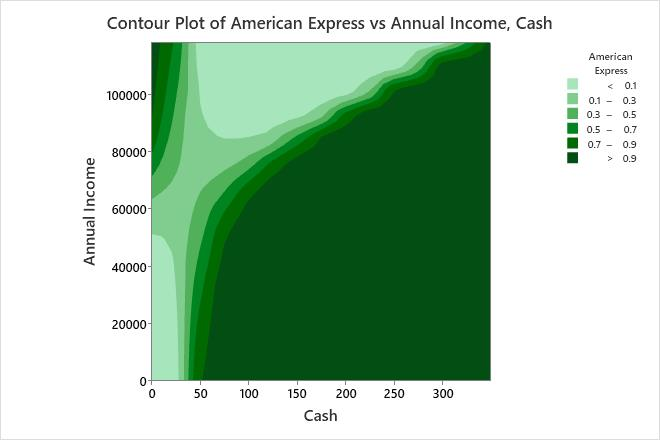# Example of Contour Plot with a binary logistic regression model

A financial analyst investigates the factors that are associated with the probability that a college student has certain credit cards. The analyst randomly samples college students for a survey. The survey asks the students questions about their education and finances.

Because the response is binary, the analyst uses binary logistic regression to determine whether the financial variables are related to the probability that the college students have an American Express credit card. The analyst creates a plot based on the binary logistic regression model to better understand the relationship between the predictors and the probability that the college students have an American Express credit card.

1. Open the sample data, CreditSurvey.MTW.
2. Choose Stat > Regression > Binary Logistic Regression > Contour Plot.
3. From Response, select American Express.
4. Under Select a pair of variables for a single plot, select Cash from X Axis and select Annual Income from Y Axis.
5. Click OK.

## Interpret the results

Minitab uses the stored model to create the contour plot. The contours have a complex shape that is difficult to describe. In general, students with more cash in their wallet and lower incomes have a higher probability of having an American Express credit card. Students with a small amount of cash have a low probability of having an American Express credit card, unless they also have a very high annual income. Students with a higher annual income have a lower probability of having an American Express credit card, unless they also have either a very low or a very high amount of cash.

The legend on the plot indicates that darker colors correspond to higher probabilities of having an American Express credit card.

###### Tip

To annotate the values of the predictors and the responses for any point on this plot, use Plant Flag. To plant a flag, right-click the plot, choose Plant Flag in the menu that appears, and then click the point on the plot that you want to annotate. Use Predict to determine whether these points are unusual and to assess the precision of the predictions.By using this site you agree to the use of cookies for analytics and personalized content.  Read our policy